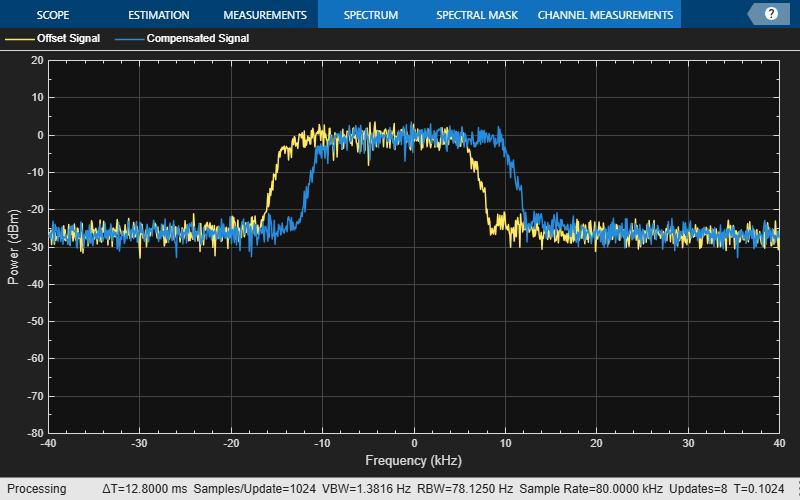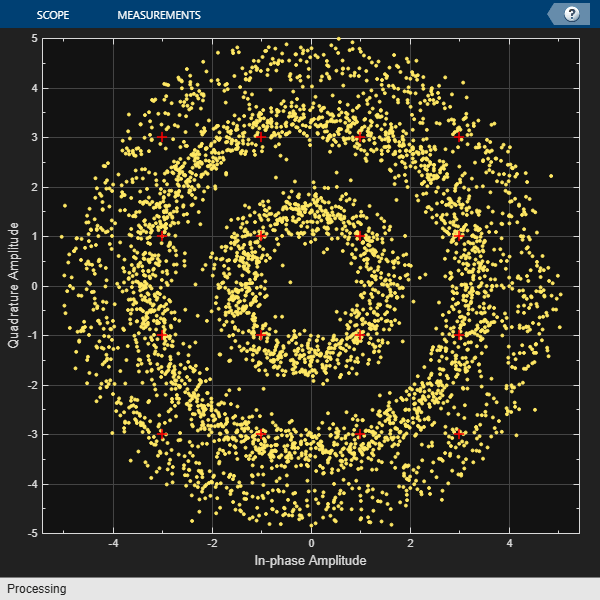comm.CoarseFrequencyCompensator

Compensate for frequency offset for PAM, PSK, or QAM

Description

The CoarseFrequencyCompensator System object™ compensates for the frequency offset of received signals.

To compensate for the frequency offset of a PAM, PSK, or QAM signal:

1. Define and set up your coarse frequency compensator object. See Construction.

2. Call step to compensate for the frequency offset of a PAM, PSK, or QAM signal according to the properties of comm.CoarseFrequencyCompensator. The behavior of step is specific to each object in the toolbox.

Note

Starting in R2016b, instead of using the step method to perform the operation defined by the System object, you can call the object with arguments, as if it were a function. For example, y = step(obj,x) and y = obj(x) perform equivalent operations.

Construction

CFC = comm.CoarseFrequencyCompensator creates a coarse frequency offset compensator object, CFC. This object uses an open-loop technique to estimate and compensate for the carrier frequency offset in a received signal.

CFC = comm.CoarseFrequencyCompensator(Name,Value) creates a coarse frequency offset compensator object, CFC, with the specified property Name set to the specified Value. You can specify additional name-value pair arguments in any order as Name1,Value1,...,NameN,ValueN.

Properties

Modulation

Modulation type

Specify the signal modulation type as BPSK, QPSK, OQPSK, 8PSK, PAM, or QAM. The default is QAM. This property is nontunable.

Algorithm

Algorithm used to estimate frequency offset

Specify the estimation algorithm as one of FFT-based or Correlation-based. The default is FFT-based. This property is nontunable.

The table shows the allowable combinations of the modulation type and the estimation algorithm.

ModulationFFT-Based AlgorithmCorrelation-Based Algorithm
BPSK, QPSK, 8PSK, PAM
OQPSK, QAM

Use the correlation-based algorithm for HDL implementations and for other situations in which you want to avoid using an FFT.

This property appears when Modulation is 'BPSK', 'QPSK', '8PSK', or 'PAM'.

FrequencyResolution

Frequency resolution (Hz)

Specify the frequency resolution for the offset frequency estimation as a positive, real scalar of data type double. This property establishes the FFT length used to perform spectral analysis and must be less than the sample rate. The default is 0.001. This property is nontunable.

MaximumFrequencyOffset

Maximum measurable frequency offset (Hz)

Specify the maximum measurable frequency offset as a positive, real scalar of data type double.

The value of this property must be less than fsamp / M, where fsamp is the sample rate and M is the modulation order. As a best practice, set MaximumOffset to less than r / (4M). This property applies only if Algorithm is Correlation-based. The default is 0.05. This property is nontunable.

SampleRate

Sample rate (Hz)

Specify the sample rate in samples per second as a positive, real scalar of data type double. The default is 1. This property is nontunable.

SamplesPerSymbol

Samples per symbol

Specify the number of samples per symbol, s, as a real positive finite integer scalar, such that s ≥ 2. The default value is 4. This property is nontunable.

This property appears when Modulation is 'OQPSK'.

Methods

 info Characteristic information about coarse frequency compensator step Compensate for frequency offset
Common to All System Objects
release

Allow System object property value changes

reset

Reset internal states of System object

Examples

collapse all

Compensate for a 4 kHz frequency offset imposed on a noisy QPSK signal.

Set the example parameters.

nSym = 2048;       % Number of input symbols
sps = 4;           % Samples per symbol
nSamp = nSym*sps;  % Number of samples
fs = 80000;        % Sampling frequency (Hz)

Create a square root raised cosine transmit filter.

txfilter = comm.RaisedCosineTransmitFilter(...
'RolloffFactor',0.2, ...
'FilterSpanInSymbols',8,   ...
'OutputSamplesPerSymbol',sps);

Create a phase frequency offset object to introduce the 4 kHz frequency offset.

freqOffset = comm.PhaseFrequencyOffset(...
'FrequencyOffset',-4000, ...
'SampleRate',fs);

Create a coarse frequency compensator object to compensate for the offset.

freqComp = comm.CoarseFrequencyCompensator(...
'Modulation','QPSK', ...
'SampleRate',fs, ...
'FrequencyResolution',1);

Generate QPSK symbols, filter the modulated data, pass the signal through an AWGN channel, and apply the frequency offset.

data = randi([0 3],nSym,1);
modData = pskmod(data,4,pi/4);
txSig = txfilter(modData);
rxSig = awgn(txSig,20,'measured');
offsetData = freqOffset(rxSig);

Compensate for the frequency offset using freqComp. When the frequency offset is high, it is beneficial to do coarse frequency compensation prior to receive filtering because filtering suppresses energy in the useful spectrum.

[compensatedData,estFreqOffset] = freqComp(offsetData);

Display the estimate of the frequency offset.

estFreqOffset
estFreqOffset =

-3.9999e+03

Return information about the freqComp object. To obtain the FFT length, you must call freqComp prior to calling the info method.

freqCompInfo = info(freqComp)
freqCompInfo =

struct with fields:

FFTLength: 131072
Algorithm: 'FFT-based'

Create a spectrum analyzer object and plot the offset and compensated spectra. Verify that the compensated signal has a center frequency at 0 Hz and that the offset signal has a center frequency at -4 kHz.

specAnal = dsp.SpectrumAnalyzer('SampleRate',fs,'ShowLegend',true, ...
'ChannelNames',{'Offset Signal' 'Compensated Signal'});
specAnal([offsetData compensatedData])Correct for a phase and frequency offset in a noisy QAM signal using a carrier synchronizer. Then correct for the offsets using both a carrier synchronizer and a coarse frequency compensator.

Set the example parameters.

fs = 10000;           % Symbol rate (Hz)
sps = 4;              % Samples per symbol
M = 16;               % Modulation order
k = log2(M);          % Bits per symbol

Create a QAM modulator and an AWGN channel.

channel = comm.AWGNChannel('EbNo',20,'BitsPerSymbol',k,'SamplesPerSymbol',sps);

Create a constellation diagram object to visualize the effects of the offset compensation techniques. Specify the constellation diagram to display only the last 4000 samples.

constdiagram = comm.ConstellationDiagram(...
'ReferenceConstellation',qammod(0:M-1,M), ...
'SamplesPerSymbol',sps, ...
'SymbolsToDisplaySource','Property','SymbolsToDisplay',4000, ...
'XLimits',[-5 5],'YLimits',[-5 5]);

Introduce a frequency offset of 400 Hz and a phase offset of 30 degrees.

phaseFreqOffset = comm.PhaseFrequencyOffset(...
'FrequencyOffset',400,...
'PhaseOffset',30,...
'SampleRate',fs);

Generate random data symbols and apply 16-QAM modulation.

data = randi([0 M-1],10000,1);
modSig = qammod(data,M);

Create a raised cosine filter object and filter the modulated signal.

txfilter = comm.RaisedCosineTransmitFilter('OutputSamplesPerSymbol',sps, ...
'Gain',sqrt(sps));
txSig = txfilter(modSig);

Apply the phase and frequency offset, and then pass the signal through the AWGN channel.

freqOffsetSig = phaseFreqOffset(txSig);
rxSig = channel(freqOffsetSig);

Apply fine frequency correction to the signal by using the carrier synchronizer.

fineSync = comm.CarrierSynchronizer('DampingFactor',0.7, ...
'NormalizedLoopBandwidth',0.005, ...
'SamplesPerSymbol',sps, ...
'Modulation','QAM');
rxData = fineSync(rxSig);

Display the constellation diagram of the last 4000 symbols.

constdiagram(rxData)Even with time to converge, the spiral nature of the plot shows that the carrier synchronizer has not yet compensated for the large frequency offset. The 400 Hz offset is 1% of the sample rate.

Repeat the process with a coarse frequency compensator inserted before the carrier synchronizer.

Create a coarse frequency compensator to reduce the frequency offset to a manageable level.

coarseSync = comm.CoarseFrequencyCompensator('Modulation','QAM','FrequencyResolution',1,'SampleRate',fs*sps);

Pass the received signal to the coarse frequency compensator and then to the carrier synchronizer.

syncCoarse = coarseSync(rxSig);
rxData = fineSync(syncCoarse);

Plot the constellation diagram of the signal after coarse and fine frequency compensation.

constdiagram(rxData)The received data now aligns with the reference constellation.

Algorithms

Correlation-Based

The correlation-based estimation algorithm, which can be used to estimate the frequency offset for PSK and PAM signals, is described in . To determine the frequency offset, Δf, the algorithm performs a maximum likelihood (ML) estimation of the complex-valued oscillation exp(j2πΔft). The observed signal, rk, is represented as

${r}_{k}={e}^{j\left(2\pi \Delta fk{\text{T}}_{s}+\theta \right)},\text{\hspace{0.17em}}1\le k\le N\text{\hspace{0.17em}},$

where Ts is the sampling interval, θ is an unknown random phase, and N is the number of samples. The maximum likelihood estimation of the frequency offset is equivalent to seeking the maximum of the likelihood function, Λ(Δf),

$\Lambda \left(\Delta f\right)\approx {|\sum _{i=1}^{N}{r}_{i}{e}^{-j2\pi \Delta fi{T}_{s}}|}^{2}=\sum _{k=1}^{N}\sum _{m=1}^{N}{r}_{k}{r}_{m}^{*}{e}^{-j2\pi \Delta f{T}_{s}\left(k-m\right)}\text{\hspace{0.17em}}.$

After simplifying, the problem is expressed as a discrete Fourier transform, weighted by a parabolic windowing function. It is expressed as

$\mathrm{Im}\left\{\sum _{k=1}^{N-1}k\left(N-k\right)R\left(k\right){e}^{j2\pi \Delta \stackrel{^}{f}{T}_{s}}\right\}=0\text{\hspace{0.17em}},$

where R(k) denotes the estimated autocorrelation of the sequence rk and is represented as

$R\left(k\right)\triangleq \frac{1}{N-k}\sum _{i=k+1}^{N}{r}_{i}\text{\hspace{0.17em}}{r}_{i-k}^{*},\text{\hspace{0.17em}}0\le k\le N-1\text{\hspace{0.17em}}.$

The term k(N–k) is the parabolic windowing function. In , it is shown that R(k) is a poor estimate of the autocorrelation of rk when k = 0 or when k is close to N. Consequently, the windowing function can be expressed as a rectangular sequence of 1s for k = 1, 2, ..., L, where LN – 1. The results is a modified ML estimation strategy in which

$\mathrm{Im}\left\{\sum _{k=1}^{L}R\left(k\right){e}^{-j2\pi \Delta \stackrel{^}{f}k{T}_{s}}\right\}=0\text{\hspace{0.17em}}.$

This results in an estimate of $\Delta \stackrel{^}{f}$ in which

$\Delta \stackrel{^}{f}\cong \frac{{f}_{samp}}{\pi \left(L+1\right)}\mathrm{arg}\left\{\sum _{k=1}^{L}R\left(k\right)\right\}\text{\hspace{0.17em}}.$

The sampling frequency, fsamp, is the reciprocal of Ts. The number of elements used to compute the autocorrelation sequence, L, are determined as

$L=\mathrm{round}\left(\frac{{f}_{samp}}{{f}_{max}}\right)-1,\text{\hspace{0.17em}}$

where fmax is the maximum expected frequency offset and round is the nearest integer function. The frequency offset estimate improves when L ≥ 7 and leads to the recommendation that fmaxfsamp / (4M).

FFT-Based

FFT-based algorithms can be used to estimate the frequency offset for all modulation types. Two variations are used in comm.CoarseFrequencyCompensator.

• For BPSK, QPSK, 8PSK, PAM, or QAM modulations the FFT-based algorithm used is described in . The algorithm estimates $\Delta \stackrel{^}{f}$ by using a periodogram of the mth power of the received signal and is given as

$\Delta \stackrel{^}{f}=\frac{{f}_{samp}}{N\cdot m}\mathrm{arg}\underset{f}{\mathrm{max}}|\sum _{k=0}^{N-1}{r}^{m}\left(k\right){e}^{-j2\pi kt/N}|,\text{ }\left(-\frac{{R}_{sym}}{2}\le f\le \frac{{R}_{sym}}{2}\right)\text{\hspace{0.17em}},$

where m is the modulation order, r(k) is the received sequence, Rsym is the symbol rate, and N is the number of samples. The algorithm searches for a frequency that maximizes the time average of the mth power of the received signal multiplied by various frequencies in the range of [–Rsym/2, Rsym/2]. As the form of the algorithm is the definition of the discrete Fourier transform of rm(t), searching for a frequency that maximizes the time average is equivalent to searching for a peak line in the spectrum of rm(t). The number of points required by the FFT is

$N={2}^{⌈{\mathrm{log}}_{2}\left(\frac{{f}_{samp}}{{f}_{r}}\right)⌉}\text{\hspace{0.17em}},$

where fr is the desired frequency resolution.

• For OQPSK modulation the FFT-based algorithm used is described in . The algorithm searches for spectral peaks at +/- 200 kHz around the symbol rate. This technique locates desired peaks in the presence of interference from spectral content around baseband frequencies due to filtering.

References

 Luise, M. and R. Regiannini. “Carrier recovery in all-digital modems for burst-mode transmissions.” IEEE® Transactions on Communications. Vol. 43, No. 2, 3, 4, Feb/Mar/April, 1995, pp. 1169–1178.

 Wang, Y., K. Shi, and E. Serpedi. “Non-Data-Aided Feedforward Carrier Frequency Offset Estimators for QAM Constellations: A Nonlinear Least-Squares Approach.” EURASIP Journal on Applied Signal Processing. 2004:13, pp. 1993–2001.

 Nakagawa, T., M. Matsui, T. Kobayashi, K. Ishihara, R. Kudo, M. Mizoguchi, and Y. Miyamoto. “Non-Data-Aided Wide-Range Frequency Offset Estimator for QAM Optical Coherent Receivers.” Optical Fiber Communication Conference and Exposition (OFC/NFOEC), 2011 and the National Fiber Optic Engineers Conference. March 2011, pp. 1–3.

 Olds, Jonathan. "Designing an OQPSK demodulator".